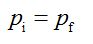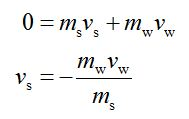# Squids are the fastest marine invertebrates, using a powerful set of muscles to take in and then eject water in a form of jet propulsion that can propel them to speeds of over 11.5 m/s. What speed (in m/s) would a stationary 1.60 kg squid achieve by ejecting 0.105 kg of water (not included in the squid's mass) at 3.00 m/s? Neglect other forces, including the drag force on the squid.

Question
1 views

Squids are the fastest marine invertebrates, using a powerful set of muscles to take in and then eject water in a form of jet propulsion that can propel them to speeds of over 11.5 m/s. What speed (in m/s) would a stationary 1.60 kg squid achieve by ejecting 0.105 kg of water (not included in the squid's mass) at 3.00 m/s? Neglect other forces, including the drag force on the squid.

check_circle

Step 1

Let the initial momentum of the water - squid system be denoted as pi and let the final momentum of the water - squid system be denoted as pf.

Let the speed of ejection of water be denoted as vw , let the speed of the squid be denoted as vs .

Let the mass of water ejected be denoted as mw , let the mass of the squid be denoted as ms.

Use the Law of conservation of momentum as follows.Step 2

As initially the water – squid system is at rest substitute 0 for pi and further express pf as,...

### Want to see the full answer?

See Solution

#### Want to see this answer and more?

Solutions are written by subject experts who are available 24/7. Questions are typically answered within 1 hour.*

See Solution
*Response times may vary by subject and question.
Tagged in